Electromagnetic Induction, AC Circuits, and Electrical Technologies

# RL Circuits

OpenStaxCollege

### Learning Objectives

• Calculate the current in an RL circuit after a specified number of characteristic time steps.
• Calculate the characteristic time of an RL circuit.
• Sketch the current in an RL circuit over time.

We know that the current through an inductor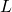cannot be turned on or off instantaneously. The change in current changes flux, inducing an emf opposing the change (Lenz’s law). How long does the opposition last? Current will flow and can be turned off, but how long does it take? [link] shows a switching circuit that can be used to examine current through an inductor as a function of time.

(a) An RL circuit with a switch to turn current on and off. When in position 1, the battery, resistor, and inductor are in series and a current is established. In position 2, the battery is removed and the current eventually stops because of energy loss in the resistor. (b) A graph of current growth versus time when the switch is moved to position 1. (c) A graph of current decay when the switch is moved to position 2.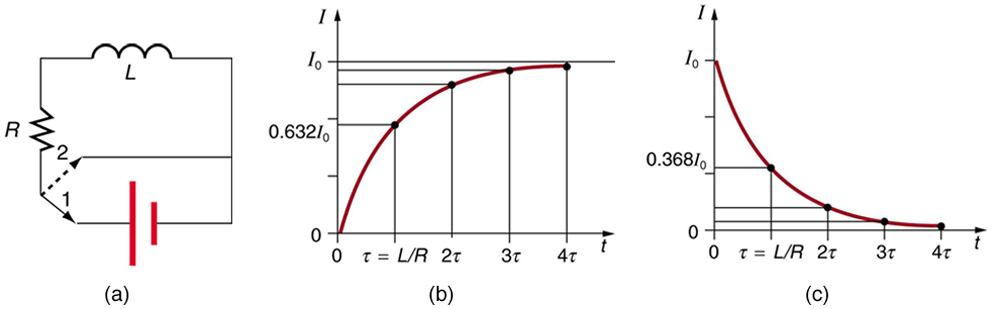When the switch is first moved to position 1 (at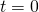), the current is zero and it eventually rises to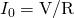, where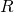is the total resistance of the circuit. The opposition of the inductoris greatest at the beginning, because the amount of change is greatest. The opposition it poses is in the form of an induced emf, which decreases to zero as the current approaches its final value. The opposing emf is proportional to the amount of change left. This is the hallmark of an exponential behavior, and it can be shown with calculus that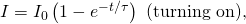is the current in an RL circuit when switched on (Note the similarity to the exponential behavior of the voltage on a charging capacitor). The initial current is zero and approacheswith a characteristic time constant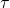for an RL circuit, given by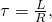wherehas units of seconds, since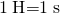.
In the first period of time, the current rises from zero to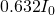, since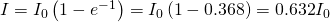. The current will go 0.632 of the remainder in the next time. A well-known property of the exponential is that the final value is never exactly reached, but 0.632 of the remainder to that value is achieved in every characteristic time. In just a few multiples of the time, the final value is very nearly achieved, as the graph in [link](b) illustrates.

The characteristic timedepends on only two factors, the inductanceand the resistance. The greater the inductance, the greateris, which makes sense since a large inductance is very effective in opposing change. The smaller the resistance, the greateris. Again this makes sense, since a small resistance means a large final current and a greater change to get there. In both cases—largeand small—more energy is stored in the inductor and more time is required to get it in and out.

When the switch in [link](a) is moved to position 2 and cuts the battery out of the circuit, the current drops because of energy dissipation by the resistor. But this is also not instantaneous, since the inductor opposes the decrease in current by inducing an emf in the same direction as the battery that drove the current. Furthermore, there is a certain amount of energy,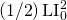, stored in the inductor, and it is dissipated at a finite rate. As the current approaches zero, the rate of decrease slows, since the energy dissipation rate is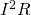. Once again the behavior is exponential, and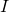is found to be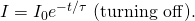(See [link](c).) In the first period of time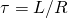after the switch is closed, the current falls to 0.368 of its initial value, since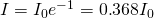. In each successive time, the current falls to 0.368 of the preceding value, and in a few multiples of, the current becomes very close to zero, as seen in the graph in [link](c).

Calculating Characteristic Time and Current in an RL Circuit

(a) What is the characteristic time constant for a 7.50 mH inductor in series with a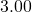resistor? (b) Find the current 5.00 ms after the switch is moved to position 2 to disconnect the battery, if it is initially 10.0 A.

Strategy for (a)

The time constant for an RL circuit is defined by.

Solution for (a)

Entering known values into the expression forgiven inyields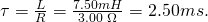Discussion for (a)

This is a small but definitely finite time. The coil will be very close to its full current in about ten time constants, or about 25 ms.

Strategy for (b)

We can find the current by using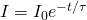, or by considering the decline in steps. Since the time is twice the characteristic time, we consider the process in steps.

Solution for (b)

In the first 2.50 ms, the current declines to 0.368 of its initial value, which is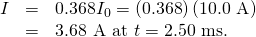After another 2.50 ms, or a total of 5.00 ms, the current declines to 0.368 of the value just found. That is,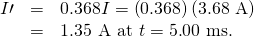Discussion for (b)

After another 5.00 ms has passed, the current will be 0.183 A (see [link]); so, although it does die out, the current certainly does not go to zero instantaneously.

In summary, when the voltage applied to an inductor is changed, the current also changes, but the change in current lags the change in voltage in an RL circuit. In Reactance, Inductive and Capacitive, we explore how an RL circuit behaves when a sinusoidal AC voltage is applied.

# Section Summary

• When a series connection of a resistor and an inductor—an RL circuit—is connected to a voltage source, the time variation of the current is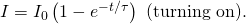where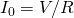is the final current.

• The characteristic time constantis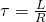, whereis the inductance andis the resistance.
• In the first time constant, the current rises from zero to, and 0.632 of the remainder in every subsequent time interval.
• When the inductor is shorted through a resistor, current decreases asHere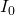is the initial current.

• Current falls to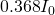in the first time interval, and 0.368 of the remainder toward zero in each subsequent time.

# Problem Exercises

If you want a characteristic RL time constant of 1.00 s, and you have a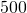resistor, what value of self-inductance is needed?

500 H

Your RL circuit has a characteristic time constant of 20.0 ns, and a resistance of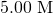. (a) What is the inductance of the circuit? (b) What resistance would give you a 1.00 ns time constant, perhaps needed for quick response in an oscilloscope?

A large superconducting magnet, used for magnetic resonance imaging, has a 50.0 H inductance. If you want current through it to be adjustable with a 1.00 s characteristic time constant, what is the minimum resistance of system?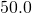Verify that after a time of 10.0 ms, the current for the situation considered in [link] will be 0.183 A as stated.

Suppose you have a supply of inductors ranging from 1.00 nH to 10.0 H, and resistors ranging from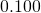to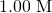. What is the range of characteristic RL time constants you can produce by connecting a single resistor to a single inductor?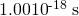to 0.100 s

(a) What is the characteristic time constant of a 25.0 mH inductor that has a resistance of? (b) If it is connected to a 12.0 V battery, what is the current after 12.5 ms?

What percentage of the final current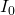flows through an inductorin series with a resistor, three time constants after the circuit is completed?

95.0%

The 5.00 A current through a 1.50 H inductor is dissipated by a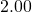resistor in a circuit like that in [link] with the switch in position 2. (a) What is the initial energy in the inductor? (b) How long will it take the current to decline to 5.00% of its initial value? (c) Calculate the average power dissipated, and compare it with the initial power dissipated by the resistor.

(a) Use the exact exponential treatment to find how much time is required to bring the current through an 80.0 mH inductor in series with a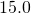resistor to 99.0% of its final value, starting from zero. (b) Compare your answer to the approximate treatment using integral numbers of. (c) Discuss how significant the difference is.

(a) 24.6 ms

(b) 26.7 ms

(c) 9% difference, which is greater than the inherent uncertainty in the given parameters.

(a) Using the exact exponential treatment, find the time required for the current through a 2.00 H inductor in series with a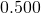resistor to be reduced to 0.100% of its original value. (b) Compare your answer to the approximate treatment using integral numbers of. (c) Discuss how significant the difference is.

## Glossary

characteristic time constant
denoted by, of a particular series RL circuit is calculated by, whereis the inductance andis the resistance